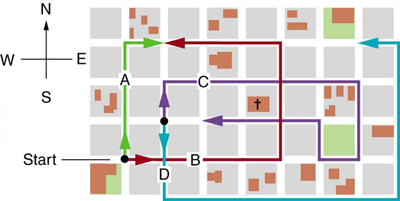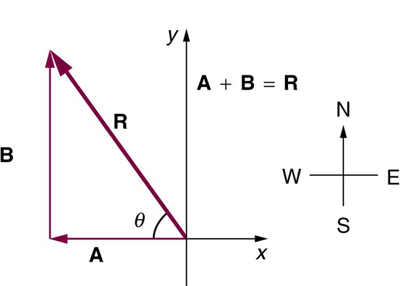# 3.3 Vector addition and subtraction: analytical methods  (Page 4/5)

 Page 4 / 5

Learn how to add vectors. Drag vectors onto a graph, change their length and angle, and sum them together. The magnitude, angle, and components of each vector can be displayed in several formats.

## Summary

• The analytical method of vector addition and subtraction involves using the Pythagorean theorem and trigonometric identities to determine the magnitude and direction of a resultant vector.
• The steps to add vectors $\mathbf{A}$ and $\mathbf{B}$ using the analytical method are as follows:

Step 1: Determine the coordinate system for the vectors. Then, determine the horizontal and vertical components of each vector using the equations

$\begin{array}{lll}{A}_{x}& =& A\phantom{\rule{0.25em}{0ex}}\text{cos}\phantom{\rule{0.25em}{0ex}}\theta \\ {B}_{x}& =& B\phantom{\rule{0.25em}{0ex}}\text{cos}\phantom{\rule{0.25em}{0ex}}\theta \end{array}$

and

$\begin{array}{lll}{A}_{y}& =& A\phantom{\rule{0.25em}{0ex}}\text{sin}\phantom{\rule{0.25em}{0ex}}\theta \\ {B}_{y}& =& B\phantom{\rule{0.25em}{0ex}}\text{sin}\phantom{\rule{0.25em}{0ex}}\theta \text{.}\end{array}$

Step 2: Add the horizontal and vertical components of each vector to determine the components ${R}_{x}$ and ${R}_{y}$ of the resultant vector, $\mathbf{\text{R}}$ :

${R}_{x}={A}_{x}+{B}_{x}$

and

${R}_{y}={A}_{y}+{B}_{y.}$

Step 3: Use the Pythagorean theorem to determine the magnitude, $R$ , of the resultant vector $\mathbf{\text{R}}$ :

$R=\sqrt{{R}_{x}^{2}+{R}_{y}^{2}}.$

Step 4: Use a trigonometric identity to determine the direction, $\theta$ , of $\mathbf{\text{R}}$ :

$\theta ={\text{tan}}^{-1}\left({R}_{y}/{R}_{x}\right).$

## Conceptual questions

Suppose you add two vectors $\mathbf{A}$ and $\mathbf{B}$ . What relative direction between them produces the resultant with the greatest magnitude? What is the maximum magnitude? What relative direction between them produces the resultant with the smallest magnitude? What is the minimum magnitude?

Give an example of a nonzero vector that has a component of zero.

Explain why a vector cannot have a component greater than its own magnitude.

If the vectors $\mathbf{A}$ and $\mathbf{B}$ are perpendicular, what is the component of $\mathbf{A}$ along the direction of $\mathbf{B}$ ? What is the component of $\mathbf{B}$ along the direction of $\mathbf{A}$ ?

## Problems&Exercises

Find the following for path C in [link] : (a) the total distance traveled and (b) the magnitude and direction of the displacement from start to finish. In this part of the problem, explicitly show how you follow the steps of the analytical method of vector addition.The various lines represent paths taken by different people walking in a city. All blocks are 120 m on a side.

(a) 1.56 km

(b) 120 m east

Find the following for path D in [link] : (a) the total distance traveled and (b) the magnitude and direction of the displacement from start to finish. In this part of the problem, explicitly show how you follow the steps of the analytical method of vector addition.

Find the north and east components of the displacement from San Francisco to Sacramento shown in [link] .

North-component 87.0 km, east-component 87.0 km

Solve the following problem using analytical techniques: Suppose you walk 18.0 m straight west and then 25.0 m straight north. How far are you from your starting point, and what is the compass direction of a line connecting your starting point to your final position? (If you represent the two legs of the walk as vector displacements $\mathbf{A}$ and $\mathbf{B}$ , as in [link] , then this problem asks you to find their sum $\mathbf{R}=\mathbf{A}+\mathbf{B}$ .)The two displacements A size 12{A} {} and B size 12{B} {} add to give a total displacement R size 12{R} {} having magnitude R size 12{R} {} and direction θ size 12{θ} {} .

Note that you can also solve this graphically. Discuss why the analytical technique for solving this problem is potentially more accurate than the graphical technique.

Repeat [link] using analytical techniques, but reverse the order of the two legs of the walk and show that you get the same final result. (This problem shows that adding them in reverse order gives the same result—that is, $\mathbf{\text{B + A = A + B}}$ .) Discuss how taking another path to reach the same point might help to overcome an obstacle blocking you other path.

30.8 m, 35.8 west of north

You drive $7\text{.}\text{50 km}$ in a straight line in a direction $15º$ east of north. (a) Find the distances you would have to drive straight east and then straight north to arrive at the same point. (This determination is equivalent to find the components of the displacement along the east and north directions.) (b) Show that you still arrive at the same point if the east and north legs are reversed in order.

Do [link] again using analytical techniques and change the second leg of the walk to $\text{25.0 m}$ straight south. (This is equivalent to subtracting $\mathbf{B}$ from $\mathbf{A}$ —that is, finding $\mathbf{\text{R}}\prime =\mathbf{\text{A – B}}$ ) (b) Repeat again, but now you first walk $\text{25}\text{.}\text{0 m}$ north and then $\text{18}\text{.}\text{0 m}$ east. (This is equivalent to subtract $\mathbf{A}$ from $\mathbf{B}$ —that is, to find $\mathbf{A}=\mathbf{B}+\mathbf{C}$ . Is that consistent with your result?)

(a) $\text{30}\text{.}\text{8 m}$ , $\text{54}\text{.}2º$ south of west

(b) $\text{30}\text{.}\text{8 m}$ , $\text{54}\text{.}2º$ north of east

A new landowner has a triangular piece of flat land she wishes to fence. Starting at the west corner, she measures the first side to be 80.0 m long and the next to be 105 m. These sides are represented as displacement vectors $\mathbf{A}$ from $\mathbf{B}$ in [link] . She then correctly calculates the length and orientation of the third side $\text{C}$ . What is her result?

You fly $\text{32}\text{.}\text{0 km}$ in a straight line in still air in the direction $35.0º$ south of west. (a) Find the distances you would have to fly straight south and then straight west to arrive at the same point. (This determination is equivalent to finding the components of the displacement along the south and west directions.) (b) Find the distances you would have to fly first in a direction $45.0º$ south of west and then in a direction $45.0º$ west of north. These are the components of the displacement along a different set of axes—one rotated $45º$ .

18.4 km south, then 26.2 km west(b) 31.5 km at $45.0º$ south of west, then 5.56 km at $45.0º$ west of north

A farmer wants to fence off his four-sided plot of flat land. He measures the first three sides, shown as $\mathbf{A},$ $\mathbf{B},$ and $\mathbf{C}$ in [link] , and then correctly calculates the length and orientation of the fourth side $\mathbf{D}$ . What is his result?

In an attempt to escape his island, Gilligan builds a raft and sets to sea. The wind shifts a great deal during the day, and he is blown along the following straight lines: $2\text{.}\text{50 km}$ $45.0º$ north of west; then $4\text{.}\text{70 km}$ $60.0º$ south of east; then $1.30\phantom{\rule{0.25em}{0ex}}\text{km}$ $25.0º$ south of west; then $5\text{.}\text{10 km}$ straight east; then $1.70\phantom{\rule{0.25em}{0ex}}\text{km}$ $5.00º$ east of north; then $7\text{.}\text{20 km}$ $55.0º$ south of west; and finally $2\text{.}\text{80 km}$ $10.0º$ north of east. What is his final position relative to the island?

$7\text{.}\text{34 km}$ , $\text{63}\text{.}5º$ south of east

Suppose a pilot flies $\text{40}\text{.}\text{0 km}$ in a direction $\text{60º}$ north of east and then flies $\text{30}\text{.}\text{0 km}$ in a direction $\text{15º}$ north of east as shown in [link] . Find her total distance $R$ from the starting point and the direction $\theta$ of the straight-line path to the final position. Discuss qualitatively how this flight would be altered by a wind from the north and how the effect of the wind would depend on both wind speed and the speed of the plane relative to the air mass.

full meaning of GPS system
how to prove that Newton's law of universal gravitation F = GmM ______ R²
sir dose it apply to the human system
prove that the centrimental force Fc= M1V² _________ r
prove that centripetal force Fc = MV² ______ r
Kaka
how lesers can transmit information
griffts bridge derivative
below me
please explain; when a glass rod is rubbed with silk, it becomes positive and the silk becomes negative- yet both attracts dust. does dust have third types of charge that is attracted to both positive and negative
what is a conductor
Timothy
hello
Timothy
below me
why below you
Timothy
no....I said below me ...... nothing below .....ok?
dust particles contains both positive and negative charge particles
Mbutene
corona charge can verify
Stephen
when pressure increases the temperature remain what?
remains the temperature
betuel
what is frequency
define precision briefly
CT scanners do not detect details smaller than about 0.5 mm. Is this limitation due to the wavelength of x rays? Explain.
hope this helps
what's critical angle
The Critical Angle Derivation So the critical angle is defined as the angle of incidence that provides an angle of refraction of 90-degrees. Make particular note that the critical angle is an angle of incidence value. For the water-air boundary, the critical angle is 48.6-degrees.
okay whatever
Chidalu
pls who can give the definition of relative density?
Temiloluwa
the ratio of the density of a substance to the density of a standard, usually water for a liquid or solid, and air for a gas.
Chidalu
What is momentum
mass ×velocity
Chidalu
it is the product of mass ×velocity of an object
Chidalu
how do I highlight a sentence]p? I select the sentence but get options like copy or web search but no highlight. tks. src
then you can edit your work anyway you want
Wat is the relationship between Instataneous velocity
Instantaneous velocity is defined as the rate of change of position for a time interval which is almost equal to zero
AstronomyByByBy Yasser IbrahimBy Tony PizurBy Zarina ChocolateBy CB BiernByBy Madison ChristianBy Brooke DelaneyBy Brooke DelaneyBy Madison ChristianBy OpenStax